## Chapter 4 triangles R.D. Sharma Solutions for Class 10th Math Exercise 4.3

Exercise 4.3

1. In a ∆ABC, AD is the bisector of ∠A, meeting side BC at D.

(i) If BD = 2.5 cm, AB = 5 cm and AC = 4.2 cm, find DC.
(ii) If BD = 2 cm, AB = 5 cm and DC = 3 cm, find AC.
(iii) If AB = 3.5 cm, AC = 4.2 cm and DC = 2.8 cm, find BD.
(iv) If AB = l0 cm, AC =14 cm and BC =6 cm, find BD and DC.
(v) If AC = 4.2 cm, DC = 6 cm and 10 cm, find AB
(vi) If AB = 5.6 cm, AC = 6 cm and DC = 3 cm, find BC.
(vii) If AD = 5.6 cm, BC = 6 cm and BD = 3.2 cm, find AC.
(viii) If AB = 10 cm, AC = 6 cm and BC = 12 cm, find BD and DC.

Solution

(i)(ii)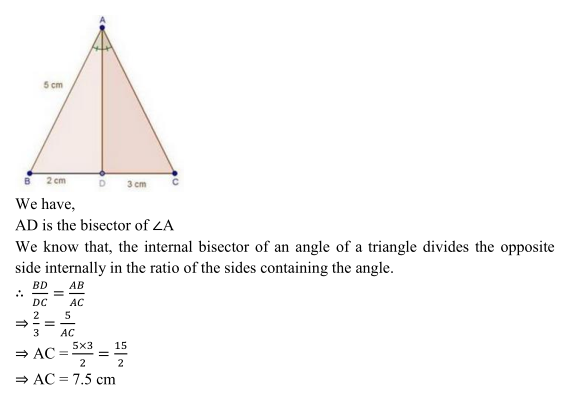(iii)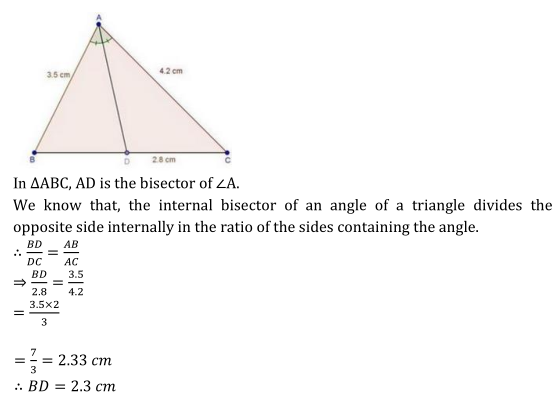(iv)(v)(vi)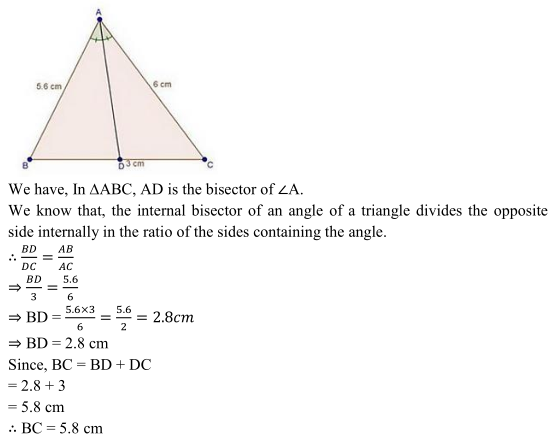(vii)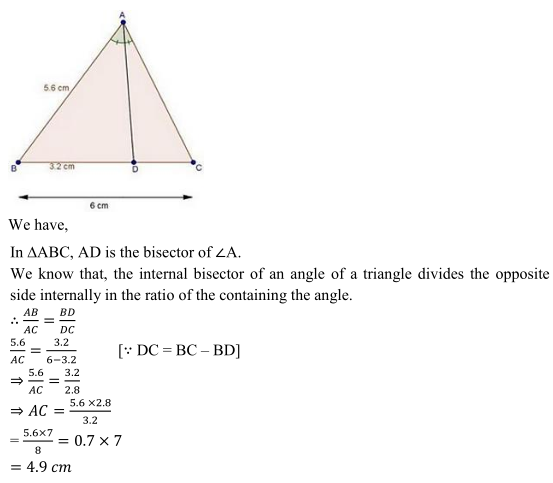(viii)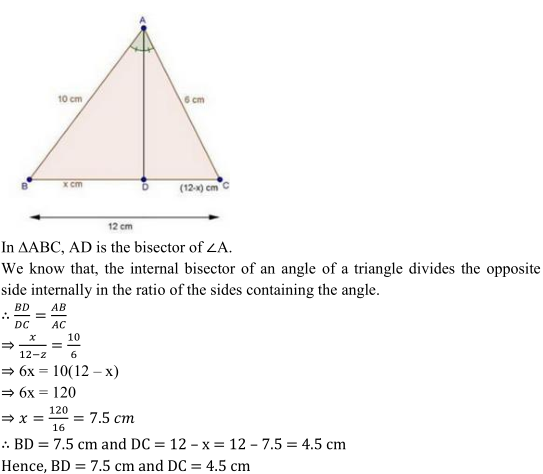2. In Fig. 4.57, AE is the bisector of the exterior ∠CAD meeting BC produced in E. If AB = 10 cm, AC = 6 cm and BC = 12 cm, and find CE .Solution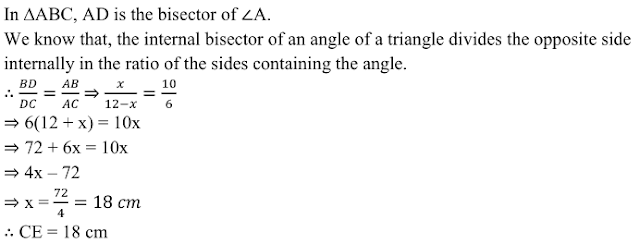3. In Fig. 4.58, △ABC is a triangle such that AB/AC = BD/DC, ∠B = 70°, ∠C = 50° . Find ∠BAD.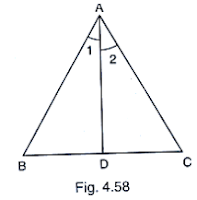Solution4. In fig. 4.59, check whether AD is the bisector of ∠A of ∆ABC in each of the following: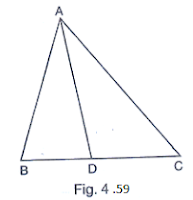(i) AB = 5cm, AC = 10cm, BD = 1.5 cm and CD = 3.5 cm
(ii) AB = 4 cm, AC = 6 cm, BD = 1.6 cm and CD = 2.4 cm
(iii) AB = 8 cm, AC = 24 cm, BD = 6 cm and BC = 24cm
(iv) AB = 6 cm, AC = 8cm, BD = l.5 cm and CD = 2 cm
(v) AB = 5 cm, AC = l2 cm, BD = 2.5 cm and BC = 9cm

Solution5. In Fig. 4.60, AD bisects ∠A, AB = 12 cm, AC = 20 cm and BD = 5 cm, determine CD.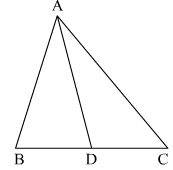Solution

It is given that AD bisects ∠A . Also, AB = 12 cm, AC = 20 cm and BD = 5 cm.
We have to find CD.
Since AD is the bisector of ∠A
12cm/20cm = 5cm/DC
12cm × Dc = 20cm × 5 cm
DC = 100/12 cm
= 8.33 cm
Hence, CD = 8.33 cm

6. In △ABC (Fig., 4.59), if ∠1 = ∠2, prove that AB/AC = BD/DC.Solution7. D, E and F are the points on sides BC, CA and AB respectively of ∆ABC such that AD bisects ∠A, BE bisects ∠B and CF bisects ∠C. If AB = 5 cm, BC = 8 cm and CA = 4 cm.Solution